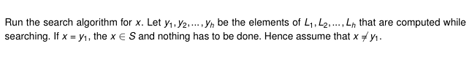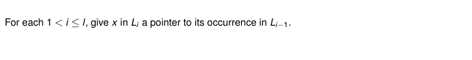# Insert into a Skip list (Algorithms)

Skip lists are randomized dictionaries which use the randomness to introduce more and more sparse versions of the original list such that parsing the lists for a certain element can be done very fast – O(log(n)) with n being the number of elements in the list.###### Example of a skip listSource: Knut Reinert

From the figure you can see that each element in the list is stored as a node with two pointers: one pointer to the same element in the level below and one pointer to the next larger element in the same level (null-pointers if these elements don't exist). Each level of a skip list starts with the element minus infinity, which isn't part of the original list we are trying to store but simply part of the architecture. Only the lowest level contains all elements of the original list. In every new level above an element of the lower list is only kept with probability p.

If we now search for a certain element (in the figure we search for the number 10) we don't have to touch every element but starting from the upper levels we follow the right pointers and skip (hence the name) all elements which only exist in lower lists, until we find an element which is bigger than the one we search for. Then we have to go down a level to refine the search, repeat the steps until we either find the element or reach the lowest list and find out that the element is not in the list.

For insertion we first search for the element and if it doesn't exist in the list yet proceed with the following steps: Flip a coin to determine in how many levels the new element has to be inserted into, from the position we found (which is necessarily on the lowest level) create nodes containing the new element pointing to itself in the level below and the next bigger element to the right while changing the previous node's pointer to the new element. If the number of positive coin flips is bigger than the current number of levels we have to introduce new minus infinity nodes and let all except the most upper one point a the new element which of course also has to point down. In the end we just have to adjust the hight variable h.

To remove an element again first search for it and then remove all nodes containing the element while adjusting the pointers. If necessary delete upper levels which became empty.

##### Get the snippets of Code for insterting into a skip list into the right order.For inserting an element x into the dictionary we proceed as follows: